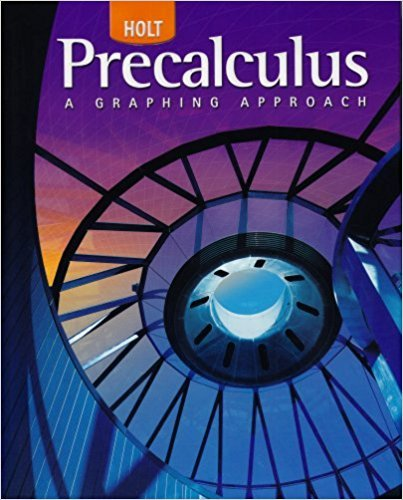×
×

# Solutions for Chapter 7.1: Graphs of the Sine, Cosine, and Tangent Functions## Full solutions for Precalculus | 1st Edition

ISBN: 9780030416477Solutions for Chapter 7.1: Graphs of the Sine, Cosine, and Tangent Functions

Solutions for Chapter 7.1
4 5 0 412 Reviews
12
2
##### ISBN: 9780030416477

Precalculus was written by and is associated to the ISBN: 9780030416477. This textbook survival guide was created for the textbook: Precalculus, edition: 1. Chapter 7.1: Graphs of the Sine, Cosine, and Tangent Functions includes 61 full step-by-step solutions. Since 61 problems in chapter 7.1: Graphs of the Sine, Cosine, and Tangent Functions have been answered, more than 26154 students have viewed full step-by-step solutions from this chapter. This expansive textbook survival guide covers the following chapters and their solutions.

Key Calculus Terms and definitions covered in this textbook
• Additive inverse of a complex number

The opposite of a + bi, or -a - bi

• Arcsecant function

See Inverse secant function.

• Arctangent function

See Inverse tangent function.

• Conic section (or conic)

A curve obtained by intersecting a double-napped right circular cone with a plane

• Discriminant

For the equation ax 2 + bx + c, the expression b2 - 4ac; for the equation Ax2 + Bxy + Cy2 + Dx + Ey + F = 0, the expression B2 - 4AC

• Eccentricity

A nonnegative number that specifies how off-center the focus of a conic is

• First-degree equation in x , y, and z

An equation that can be written in the form.

• Focal width of a parabola

The length of the chord through the focus and perpendicular to the axis.

• Lemniscate

A graph of a polar equation of the form r2 = a2 sin 2u or r 2 = a2 cos 2u.

• Linear inequality in x

An inequality that can be written in the form ax + b < 0 ,ax + b … 0 , ax + b > 0, or ax + b Ú 0, where a and b are real numbers and a Z 0

• Linear regression line

The line for which the sum of the squares of the residuals is the smallest possible

• Minor axis

The perpendicular bisector of the major axis of an ellipse with endpoints on the ellipse.

• Origin

The number zero on a number line, or the point where the x- and y-axes cross in the Cartesian coordinate system, or the point where the x-, y-, and z-axes cross in Cartesian three-dimensional space

• Orthogonal vectors

Two vectors u and v with u x v = 0.

• Parameter

See Parametric equations.

• Quotient rule of logarithms

logb a R S b = logb R - logb S, R > 0, S > 0

• Relevant domain

The portion of the domain applicable to the situation being modeled.

• Second-degree equation in two variables

Ax 2 + Bxy + Cy2 + Dx + Ey + F = 0, where A, B, and C are not all zero.

• Standard representation of a vector

A representative arrow with its initial point at the origin

• Vertices of a hyperbola

The points where a hyperbola intersects the line containing its foci.

×